• 领接表
千次阅读
2018-12-09 20:49:33

一 . 邻接矩阵

图的邻接矩阵存储方式是用两个数组来表示图，一个一维数组来存储顶点信息，一个二维数组存储图中的边或弧的信息

数据类型

#define MAXVEX 100 /* 最大顶点数，应由用户定义 */

#define INFINITY 65535

typedef int Status;	/* Status是函数的类型,其值是函数结果状态代码，如OK等 */

typedef char VertexType; /* 顶点类型应由用户定义  */

typedef int EdgeType; /* 边上的权值类型应由用户定义 */

typedef struct

{

VertexType vexs[MAXVEX]; /* 顶点表 */

EdgeType arc[MAXVEX][MAXVEX];/* 邻接矩阵，可看作边表 */

int numNodes, numEdges; /* 图中当前的顶点数和边数  */

}MGraph;

存储过程：

/* 建立无向网图的邻接矩阵表示 */

void CreateMGraph(MGraph *G)

{

int i,j,k,w;

printf("输入顶点数和边数:\n");

scanf("%d,%d",&G->numNodes,&G->numEdges); /* 输入顶点数和边数 */

for(i = 0;i <G->numNodes;i++) /* 读入顶点信息,建立顶点表 */

scanf(&G->vexs[i]);

for(i = 0;i <G->numNodes;i++)

for(j = 0;j <G->numNodes;j++)

G->arc[i][j]=INFINITY;	/* 邻接矩阵初始化 */

for(k = 0;k <G->numEdges;k++) /* 读入numEdges条边，建立邻接矩阵 */

{

printf("输入边(vi,vj)上的下标i，下标j和权w:\n");

scanf("%d,%d,%d",&i,&j,&w); /* 输入边(vi,vj)上的权w */

G->arc[i][j]=w;

G->arc[j][i]= G->arc[i][j]; /* 因为是无向图，矩阵对称 */

}

}

二 . 领接表

图的领接表由边表（用单链表构成）和顶点表（由一维数组构成）组成，这种创建方法类似树的孩子表示法。

数据类型：

#define MAXVEX 100 /* 最大顶点数,应由用户定义 */

typedef int Status;	/* Status是函数的类型,其值是函数结果状态代码,如OK等 */

typedef char VertexType; /* 顶点类型应由用户定义 */

typedef int EdgeType; /* 边上的权值类型应由用户定义 */

typedef struct EdgeNode /* 边表结点  */

{

int adjvex;    /* 邻接点域,存储该顶点对应的下标 */

EdgeType info;		/* 用于存储权值,对于非网图可以不需要 */

struct EdgeNode *next; /* 链域,指向下一个邻接点 */

}EdgeNode;

typedef struct VertexNode /* 顶点表结点 */

{

VertexType data; /* 顶点域,存储顶点信息 */

EdgeNode *firstedge;/* 边表头指针 */

typedef struct

{

int numNodes,numEdges; /* 图中当前顶点数和边数 */



创建过程：

void  CreateALGraph(GraphAdjList *G)

{

int i,j,k;

EdgeNode *e;

printf("输入顶点数和边数:\n");

scanf("%d,%d",&G->numNodes,&G->numEdges); /* 输入顶点数和边数 */

for(i = 0;i < G->numNodes;i++) /* 读入顶点信息,建立顶点表 */

{

scanf(&G->adjList[i].data); 	/* 输入顶点信息 */

G->adjList[i].firstedge=NULL; 	/* 将边表置为空表 */

}

for(k = 0;k < G->numEdges;k++)/* 建立边表 */

{

printf("输入边(vi,vj)上的顶点序号:\n");

scanf("%d,%d",&i,&j); /* 输入边(vi,vj)上的顶点序号 */

e=(EdgeNode *)malloc(sizeof(EdgeNode)); /* 向内存申请空间,生成边表结点 */

e->adjvex=j;					/* 邻接序号为j */

e->next=G->adjList[i].firstedge;	/* 将e的指针指向当前顶点上指向的结点 */

G->adjList[i].firstedge=e;		/* 将当前顶点的指针指向e */

e=(EdgeNode *)malloc(sizeof(EdgeNode)); /* 向内存申请空间,生成边表结点 */

e->adjvex=i;					/* 邻接序号为i */

e->next=G->adjList[j].firstedge;	/* 将e的指针指向当前顶点上指向的结点 */

G->adjList[j].firstedge=e;		/* 将当前顶点的指针指向e */

}

}

两种方法的区别：邻接矩阵时间复杂度为O(N2) 边数的平方 , 邻接表时间复杂度O（N+E）顶点数+边数

对于边少顶点多（稀疏图）使用领接表创建，边多顶点少（稠密图）用邻接矩阵创建。

更多相关内容
• 题目详情： 答案完整代码： #include <stdio.h> #include <stdlib.h>...#define MVNum 100 //最大顶点数 typedef struct ArcNode{ //结点 int adjvex; //邻接点的位置 struct .

### 题目详情：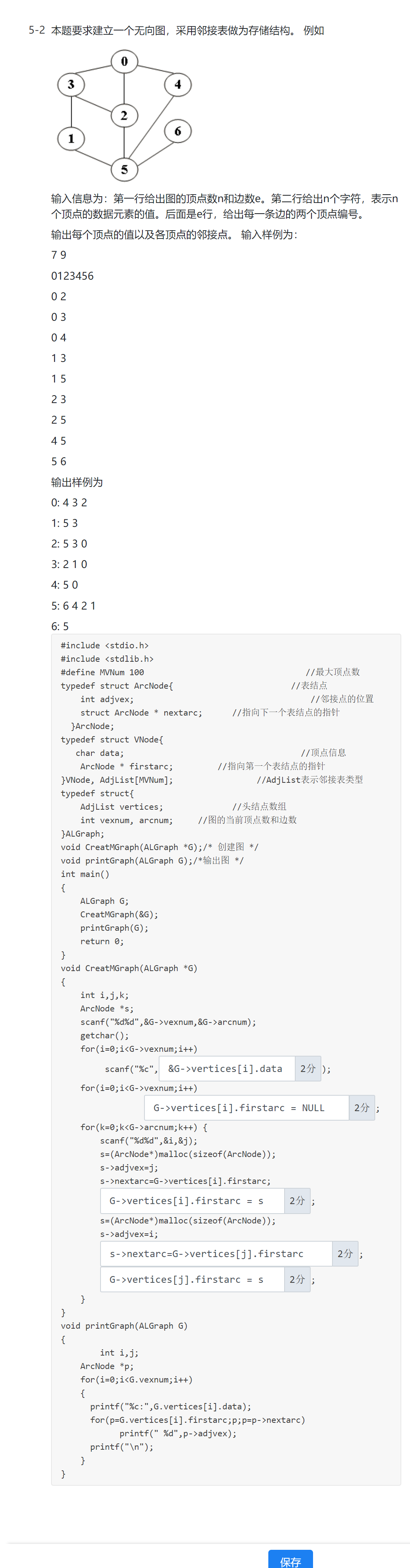### 答案完整代码：

#include <stdio.h>
#include <stdlib.h>
#define MVNum 100                                 //最大顶点数
typedef struct ArcNode{                        //表结点
struct ArcNode * nextarc;      //指向下一个表结点的指针
}ArcNode;
typedef struct VNode{
char data;                                    //顶点信息
ArcNode * firstarc;         //指向第一个表结点的指针
typedef struct{
int vexnum, arcnum;     //图的当前顶点数和边数
}ALGraph;
void CreatMGraph(ALGraph *G);/* 创建图 */
void printGraph(ALGraph G);/*输出图 */
int main()
{
ALGraph G;
CreatMGraph(&G);
printGraph(G);
return 0;
}
void CreatMGraph(ALGraph *G)/*创建图*/
{
int i,j,k;
ArcNode *s;
scanf("%d%d",&G->vexnum,&G->arcnum);
getchar();
for(i=0;i<G->vexnum;i++)
scanf("%c",&G->vertices[i].data);
for(i=0;i<G->vexnum;i++)

G->vertices[i].firstarc = NULL
;
for(k=0;k<G->arcnum;k++) {
scanf("%d%d",&i,&j);
s=(ArcNode*)malloc(sizeof(ArcNode));
s->nextarc=G->vertices[i].firstarc;

G->vertices[i].firstarc = s;
s=(ArcNode*)malloc(sizeof(ArcNode));

s->nextarc=G->vertices[j].firstarc;

G->vertices[j].firstarc = s;
}
}
void printGraph(ALGraph G)/*输出图*/
{
int i,j;
ArcNode *p;
for(i=0;i<G.vexnum;i++)
{
printf("%c:",G.vertices[i].data);
for(p=G.vertices[i].firstarc;p;p=p->nextarc)
printf("\n");
}
}
展开全文数据结构
• 20171124  图的概念：    图的基本性质：  无向图：  有向图：  连通图：  图的权：有些图的边或者狐剧有与他相关的数字，这种与图的边或者狐相关的数叫做权... 图的存储结构-邻接  无向图的邻接：  有向

20171124

图的概念：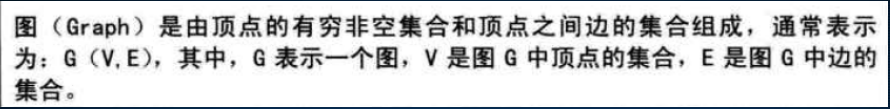图的基本性质：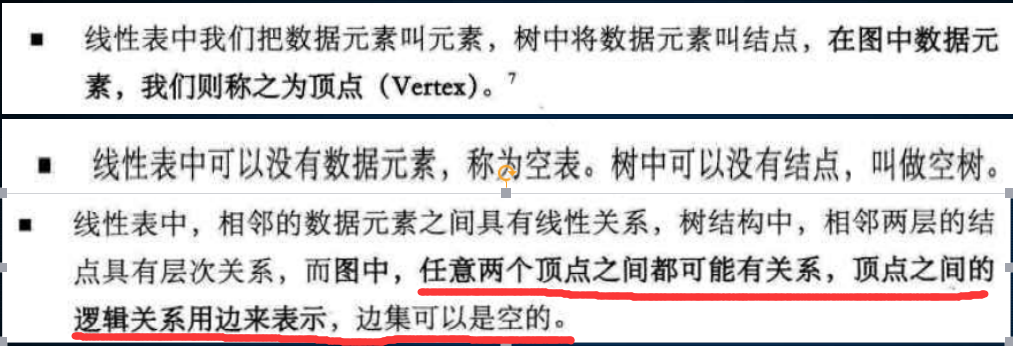无向图：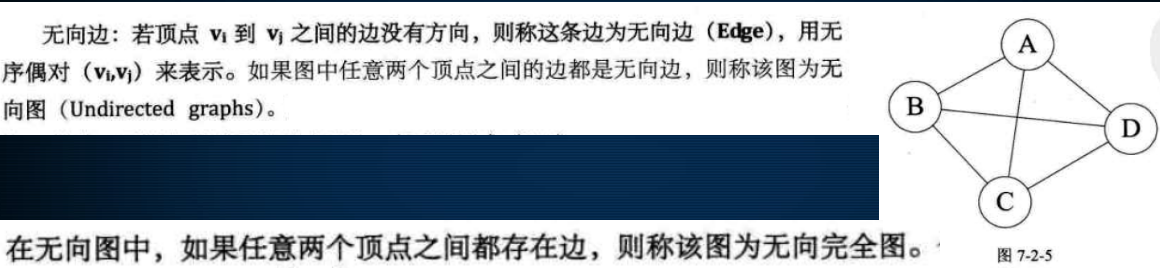有向图：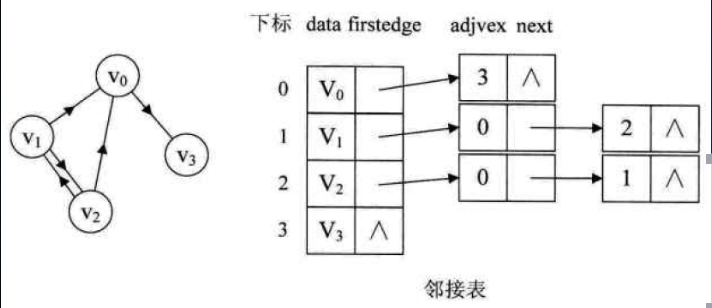连通图：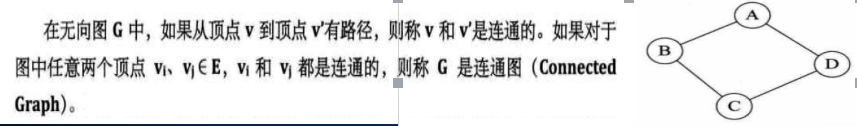图的权：有些图的边或者狐剧有与他相关的数字，这种与图的边或者狐相关的数叫做权。

图的度：无向图顶点的边数叫度，有向图顶点的边数叫出度和入度 。

图的数据存储结构-邻接矩阵：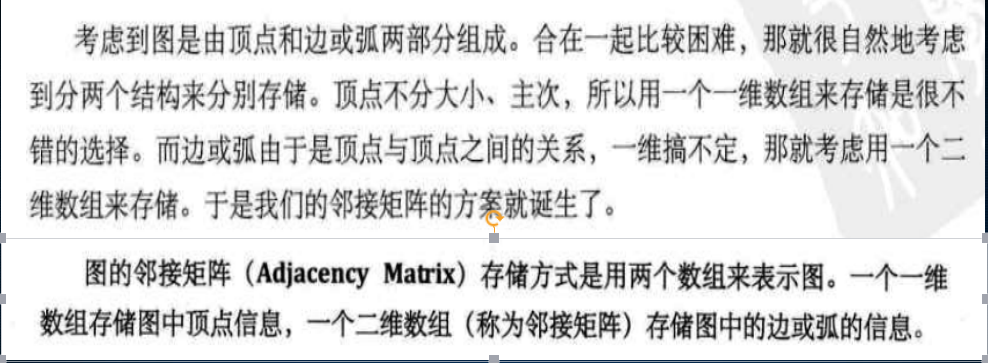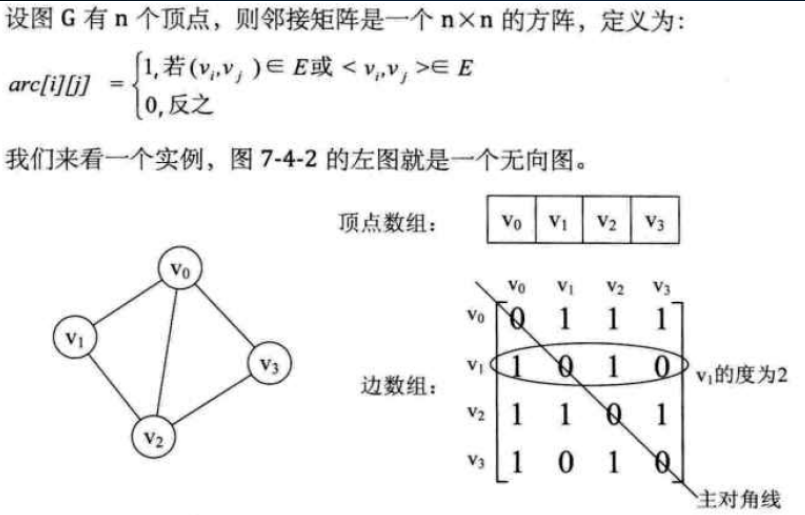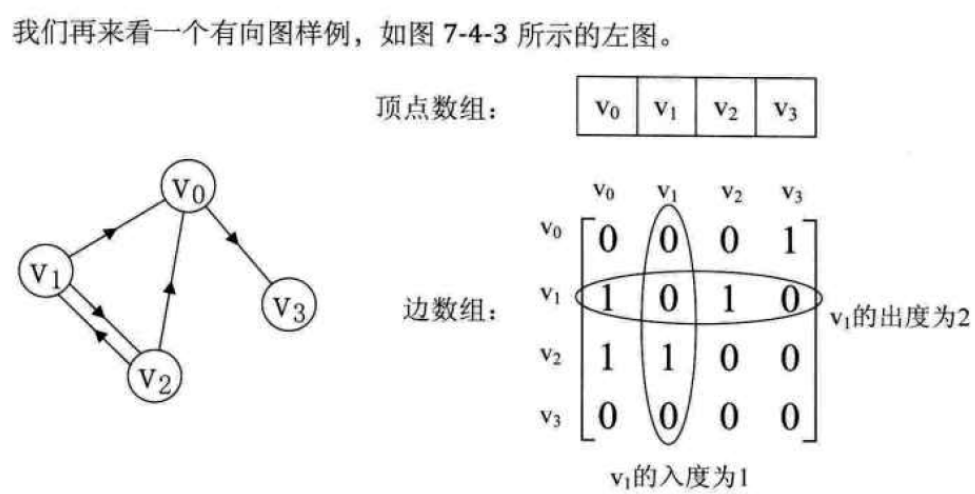带权邻接矩阵表示法：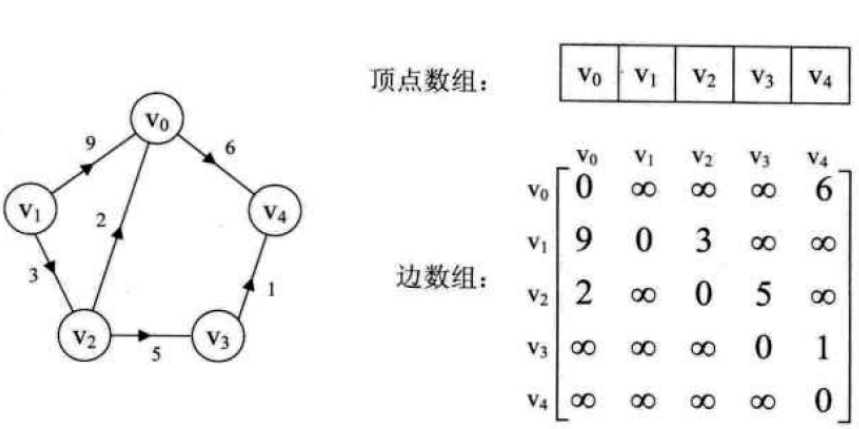图的存储结构-邻接表

无向图的邻接表：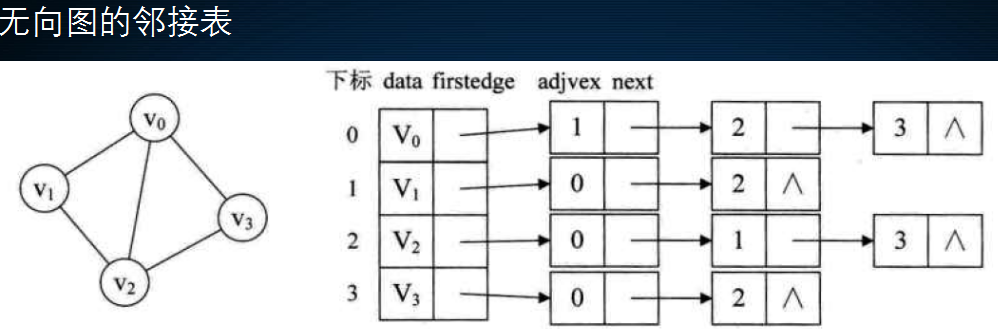有向图的邻接表：逆邻接表：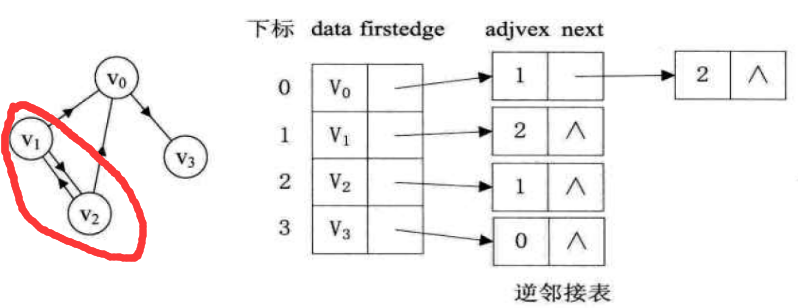带权邻接表：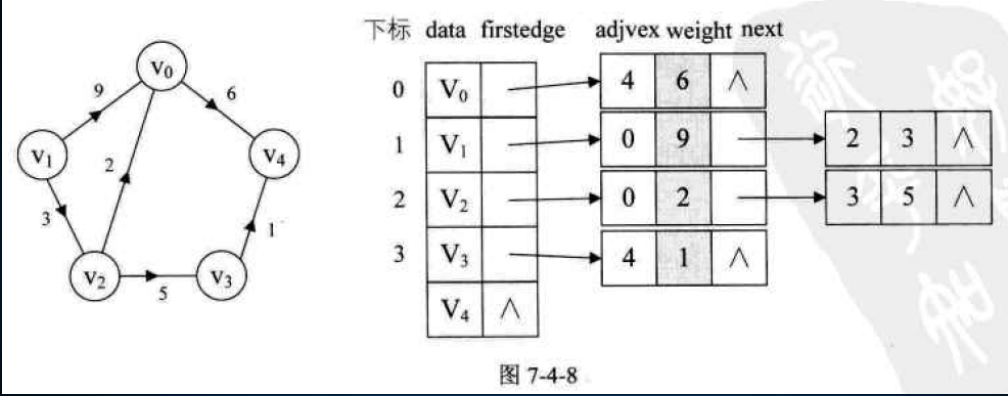展开全文• #include using namespace std; #define MAX_NODE 30 #define MAX_INFO 10 bool isOutput[MAX_NODE]; //记录该节点有没有输出 struct ListNode { ListNode(){next=NULL;} int position; ...stru
#include<iostream>
using namespace std;

#define MAX_NODE 30
#define MAX_INFO 10
bool isOutput[MAX_NODE];   //记录该节点有没有输出
struct ListNode
{
ListNode(){next=NULL;}
int position;
ListNode* next;
};
struct VertexNode
{
VertexNode()
{
inDegree=0;
}
int currentPosition;		//当前节点在图存储结构中数组的位置
int inDegree;				//该节点的入度
char info[MAX_INFO];		//该节点的信息
};
struct Graphic
{
int vertex,edge;
VertexNode vers[MAX_NODE];
};

void createGraphic(Graphic& graphic)
{

cout<<"请输入节点数和边数: ";
cin>>graphic.vertex>>graphic.edge;

cout<<"请输入节点信息:"<<endl;
int i;
for(i=0;i<graphic.vertex;i++)
{
cout<<"请输入第"<<i<<"个节点信息:";
cin>>graphic.vers[i].info;

}

cout<<"请输入边信息:"<<endl;

int first=0;
int second=0;
for(i=0;i<graphic.edge;i++)
{
cout<<"请输入第"<<i<<"条边信息:";
cin>>first>>second;
ListNode* temp=new ListNode;
temp->position=second;
++graphic.vers[second].inDegree;
}

for(i=0;i<graphic.vertex;i++)
{
isOutput[i]=false;
}

for(i=0;i<graphic.vertex;i++)
{
graphic.vers[i].currentPosition=i;
}
}

void printGraphic(Graphic graphic)
{
int i;
ListNode* temp=NULL;
for(i=0;i<graphic.vertex;i++)
{
cout<<graphic.vers[i].info<<"--->";
if(!temp)
{
cout<<"NULL";
}
while(temp)
{
cout<<temp->position<<" ";
temp=temp->next;
}
cout<<endl;
}

for(i=0;i<graphic.vertex;i++)
{
cout<<"节点"<<i<<"的入度:";
cout<<graphic.vers[i].inDegree<<endl;
}
cout<<endl;
}
VertexNode* findZeroInDegreeNode(Graphic& graphic)
{
int i;
for(i=0;i<graphic.vertex;i++)
{
if(graphic.vers[i].inDegree==0)
{
graphic.vers[i].inDegree=1;
return &graphic.vers[i];
}
}
return NULL;
}

void TopologicalSortForVertex(Graphic& graphic,int position)
{
{
{
}
}
}
void TopologicalSort(Graphic& graphic)
{
VertexNode* zeroNode=findZeroInDegreeNode(graphic);
if(!zeroNode)
return;
if(!isOutput[zeroNode->currentPosition])
{
cout<<zeroNode->info<<" ";
isOutput[zeroNode->currentPosition]=true;
}

TopologicalSortForVertex(graphic,zeroNode->currentPosition);
TopologicalSort(graphic);
}
void main()
{
Graphic myGraphic;
createGraphic(myGraphic);
printGraphic(myGraphic);
TopologicalSort(myGraphic);
}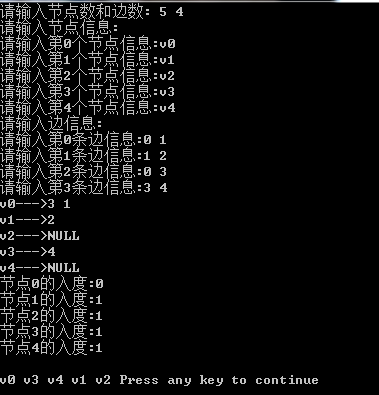展开全文• /* 将边置为空 */ } for(i=0;i;i++) /* 建立边 */ { for(j=0;j;j++) { if (G.arc[i][j]==1) { e=(EdgeNode *)malloc(sizeof(EdgeNode)); e->adjvex=j; /* 邻接序号为j */ e...有向图 无向图 领接表 广度优先
• ## 无向图的领接表

千次阅读 2016-07-27 16:32:55
无向图的领接表。 #include #include typedef struct EdgeNode{ // 边表结点 int adjvex; // 领接点域，存储该顶点对应的下标 struct EdgeNode *next; // 链域，指向下一个领接点 }EdgeNode; typedef ...
• 采用邻接来实现图的存储，并输入输出邻接的信息，并用邻接来实现图的广度优先遍历。
•数据结构
• 头结点里放当前顶点的值，以及它第一条弧的指针，结点放邻接点的序号adjvex和指向下一边的指针 网的话，有权值，增加一个成员 无向图的邻接，位置可互换，不唯一：边的顺序可以变 ...数据结构
• 邻接 主要适用于 点较多而边较少的情况。可以减少占用空间 package 图; import java.util.Scanner; public class AdjacencyList {//实现 邻接 static class Node{ char data;//结点数据 int index;//邻接...
• C 语言实现邻接Dijkstra算法求最短路径： #include<stdio.h> #include<stdlib.h> #define MAXLEN 20 #define INFINE 99999 typedef struct ArcNode //定义结构体 { int adjvex;//邻接顶点下标 ...数据结构
• ## 邻接表

千次阅读 2021-03-19 18:57:39
因此在处理稀疏图时，可以采用下面将要介绍的邻接 无向图的邻接 有向图的邻接 网图的邻接 邻接存储有向图的类 有向图邻接的构造函数初始化操作 邻接的构造函数和输出函数代码实现 #...
• ## 邻接表和逆邻接表

万次阅读 多人点赞 2019-09-16 14:39:31
邻接作为图的一种存储方式，在存储稀疏图上相对于邻接矩阵有相当大的空间节省。如一个稀疏图的顶点个个数为n，边数为e。用邻接矩阵存储需要n^2空间，而真正进行存储的只有2e个空间， 剩下的n^2-2e都浪费了。但是...
• 每条边保存一次即可，只记录发出的弧，每一条边都有一个边结点 出度：顺着单链表统计边结点 ...逆邻接：入度的邻接，数结点有几个，就入度多少 邻接结构定了，图就唯一了 ...数据结构
• 什么是邻接? 邻接（Adjacency List）是图的一种顺序存储与链式存储结合的存储方法。 对于图G中的每个顶点Vi，将所有邻接于Vi的顶点Vj链成一个单链表，这个单链表就称为顶点Vi的邻接，再将所有顶点的邻接...数据结构 邻接表
• ## 邻接矩阵和邻接表

千次阅读 2020-07-05 19:30:41
图的存储结构主要分两种，一种是邻接矩阵，一种是邻接。 1.邻接矩阵 邻接矩阵的存储方式是用两个数组来表示图。一个一维数组储存图中顶点信息，一个二维数组储存图中的边或弧的信息。 无向图 这里右图是把...数据结构
• 邻接的定义 肝不动了睡觉去了。。。 邻接的优缺点 邻接的存储结构 邻接的代码实现邻接表 广度优先遍历 深度优先遍历 数据结构
• //初始化 领接矩阵 //空出G[],便于记录 for(i = 1; i ; i++) { for(j =1 ; j ; j++) G[i][j] = 0; } //赋值 for(k = 1; k ; k++) { printf("请输入之间有路径的两个顶点vi , vj"); printf("\n...
• 文章目录前言一、十字链1.画邻接2.增加弧节点的域3.自己指向自己二、邻接多重1.画顶点2.画边3.自己指向自己 前言 近期一直在构建算法技能树的数据，借此机会，重新把数据结构与算法又温习了一遍，在看到十字...数据结构
• ## 无向图邻接表实现

千次阅读 2022-04-21 23:24:49数据结构
• 用两个数组分别储存顶点和邻接矩阵​​​​​​​ 构造无向网的邻接矩阵算法 邻接 定义存储结构节点类型 构造邻接算法 邻接与邻接矩阵间的关系 ...数据结构
• #include <stdio.h> #include <...typedef struct ArcNode//边 { int adjvex;//存储的是该顶点在顶点数组即AdjList[]中的位置 int weight; struct ArcNode *next; }ArcNode; typedef s...数据结构
• 本节主要讲述图的存储实现之二，邻接的实现和算法。邻接表
• ## 邻接表创建有向图

千次阅读 2021-11-10 11:18:58
使用邻接创建有向图，可以使用数组+链表的方式创建 #include <iostream> using namespace std; //采用邻接存储节点 #define MaxSize 10 // 定义边 typedef struct arc_node { int adj_vex; //该边所...数据结构
• 邻接实现图 图的表示方式有两种： 邻接矩阵（Adjacency Matrix） 邻接（Adjacency List） 本文采用类似邻接的方式实现图。 图的基础接口 public interface Graph<V, E> { int verticesSize(); // ...java 数据结构 邻接表
• 图的邻接存储结构 一般来说，图更多的是采用链表存储，具体的存储方法有 3 种，分别是邻接、邻接多重和十字链 本篇文章将优先介绍邻接！！！ 邻接点：在图中，如果两个点相互连通，且通过其中一个顶点...数据结构
• 邻接1.无向图2.有向图3.网图4. 邻接的建立5. 邻接的优劣三. 邻接矩阵与邻接的关系 一. 邻接矩阵 数组表示法 1. 无向图 图的邻接矩阵(Adjacency Matrix)存储方式是用两个数组来表示图。一个一维数组存储图中...数据结构...